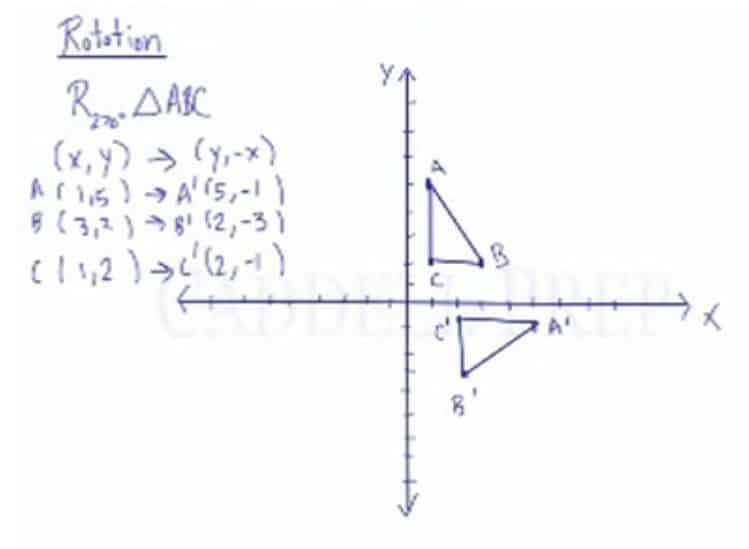In this video, you will learn how to do a rotation graphically and numerically, using the coordinates.

Rotations notations are commonly expressed as
R90, R180, and R270, where the rotation is always counterclockwise.

Rotations in the clockwise direction corresponds to rotations in the counterclockwise direction:
R-90 = R270,
R-180 = R180,
and R-270 = R90

Rotation Notations:
R90 (x,y) = (-y,x),
R180 (x,y) = (-x,-y),
and R270 (x,y) = (y,-x),

Apply a rotation of 270 degrees to triangle ABC with points A(1,5), B(3,2), and C(1,2).

Identify the appropriate rotation notation
R270 (x,y) = (y,-x),

Perform the operation within the notation to each coordinate point
A(1,5)$\rightarrow$ A'(-5,1)
B(3,2)$\rightarrow$ B'(-2,3)
C(1,2)$\rightarrow$ C'(-2,1)

Now, we have the points of the image after the transformation:
A'(-5,1), B'(-2,3), and C'(-2,1)## Video-Lesson Transcript

Let’s talk about rotation on the$xy$ coordinate plane.

We’ll cover rotations of$90^{\circ}$,$180^{\circ}$, and$270^{\circ}$.

First of all, whenever we say rotation of a positive angle, it always means counterclockwise. The hands of a clock move this way, counterclockwise means opposite of the clock. So, counterclockwise is the other direction.

The$x$-axis and$y$-axis is perpendicular to each other. That means the angle between them is$90^{\circ}$.

Rotation of$90^{\circ}$, we move this triangle from this quadrant or area into the next quadrant.

Rotation of$180^{\circ}$ is going into two quadrants.$270^{\circ}$ will go into three quadrants.

And we don’t do$360^{\circ}$ because it’s right back where we started. So, don’t worry about rotating$360^{\circ}$ because we’ll end exactly where we started.

You might also see rotations for$-90^{\circ}$, rotations of$-180^{\circ}$, and rotations of$-270^{\circ}$.

If$90^{\circ}$ is counterclockwise, then$-90^{\circ}$ is clockwise direction.$-90^{\circ}$ is the same as$270^{\circ}$.$-180^{\circ}$ is the same as$180^{\circ}$.

And rotating$-270^{\circ}$ is the same as$90^{\circ}$.

Now that we have an idea of what quadrant we’d end up in, let’s take a look at the specific rules that tells exactly where each coordinate will go.$R_{90} (x, y) \rightarrow (-y, x)$$R_{180} (x, y) \rightarrow (-x, -y)$$R_{270} (x, y) \rightarrow (y, -x)$

Let’s try rotating this triangle$90^{\circ}$.$R_{90} \triangle {ABC}$

Let’s first write down what the rule is.$R_{90} (x, y) \rightarrow (-y, x)$$A (1, 5) \rightarrow A^\prime (-5, 1)$$B (3, 2) \rightarrow B^\prime (-2, 3)$$C (1, 2) \rightarrow C^\prime (-2, 1)$

What happened is that the$x$ coordinate of$A$ became the$y$ coordinate of$A^\prime$ and the$y$ coordinate became the$x$ coordinate after we negated it.

Let’s graph this and then draw our triangle.

Now, we have the image of triangle$ABC$ when we rotate it$90^{\circ}$.

Next, let’s rotate triangle$ABC$$180^{\circ}$.

Let’s write the rule.$R_{180} (x, y) \rightarrow (-x, -y)$

So, we’ll just get the coordinates and negate them.$A (1, 5) \rightarrow A^\prime (-1, -5)$$B (3, 2) \rightarrow B^\prime (-3, -2)$$C (1, 2) \rightarrow C^\prime (-1, -2)$

Now, let’s graph and draw our new triangle.

And now we have the image of triangle$ABC$ rotated$180^{\circ}$.

Now, let’s rotate triangle$ABC$$270^{\circ}$.

Let’s write the rule we’ll follow.$R_{270} (x, y) \rightarrow (y, -x)$

We’ll take the$y$ coordinate and then make it the$x$ coordinate then take$x$ make it negative and make it the$y$ coordinate.$A (1, 5) \rightarrow A^\prime (5, -1)$$B (3, 2) \rightarrow B^\prime (2, -3)$$C (1, 2) \rightarrow C^\prime (2, -1)$

Then let’s plot these and draw a triangle.

And now we have the image of triangle$ABC$ when rotated$270^{\circ}$.